# 11 Modeling in R

The purpose of a statistical model is to help understand what variables might best predict a phenomenon of interest, which ones have more or less influence, define a predictive equation with coefficients for each of the variables, and then apply that equation to predict values using the same input variables for other areas. This process requires samples with observations of the explanatory (or independent) and response (or dependent) variables in question.

We’ll start with a simple linear model using our Sierra February climate data to model temperature (response variable) as predicted by elevation (explanatory variable):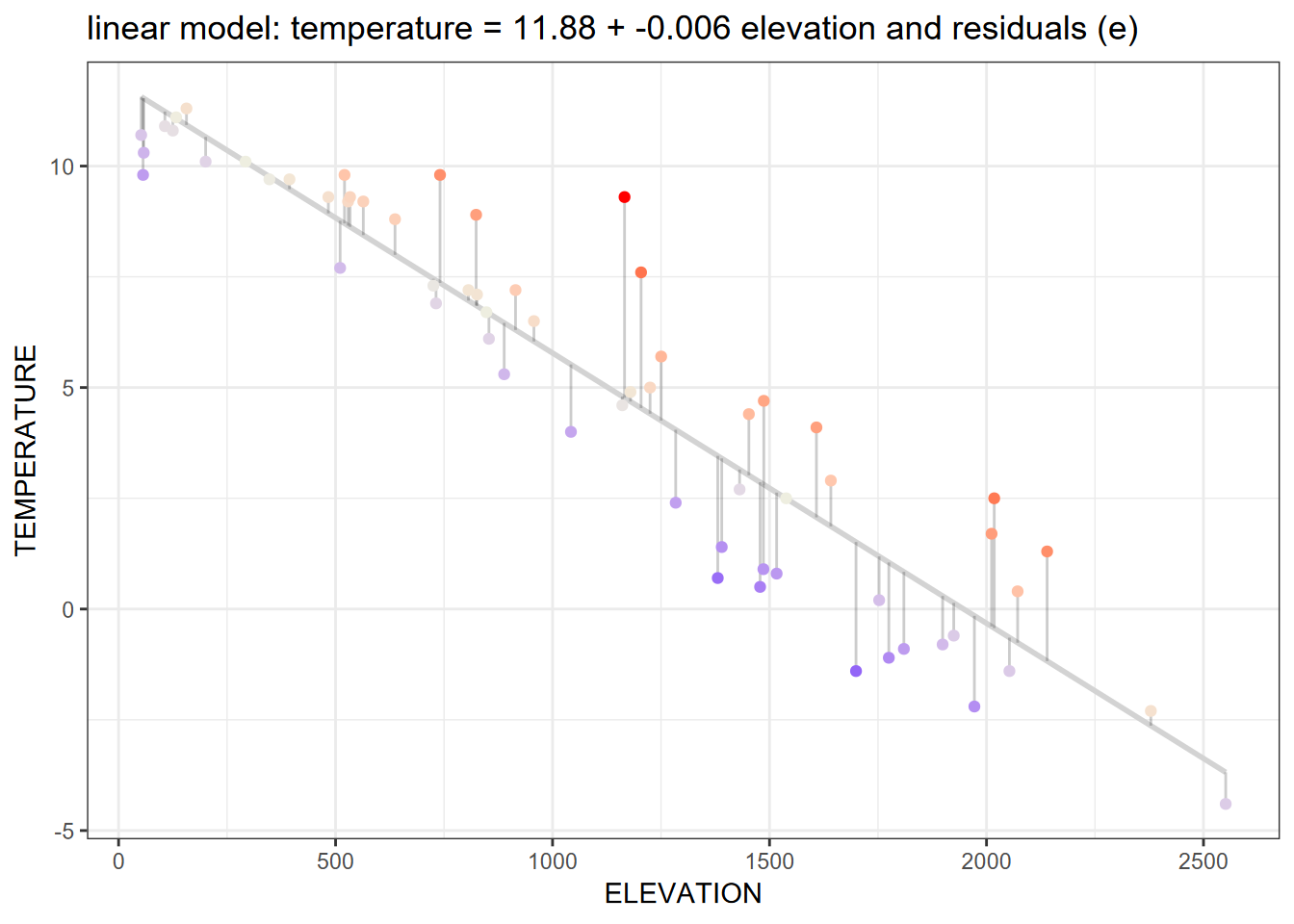Figure 11.1: Linear model

## 11.1 Some common statistical models

There are many types of statistical models. Variables may be nominal (categorical) or interval/ratio data. You may be interested in predicting a continuous interval/ratio variable from other continuous variables, or predicting the probability of an occurrence (e.g. of a species), or maybe the count of something (also maybe a species). You may be needing to classify your phenomena based on continuous variables. Here are some examples:

• lm(y ~ x) linear regression model with one explanatory variable
• lm(y ~ x1 + x2 + x3) multiple regression, a linear model with multiple explanatory variables
• glm(y ~ x, family = poisson) generalized linear model, poisson distribution; see ?family to see those supported, including binomial, gaussian, poisson, etc.
• glm(y ~ x + y, family = binomial) glm for logistic regression
• aov(y ~ x) analysis of variance (same as lm except in the summary)
• gam(y ~ x) generalized additive models
• tree(y ~ x) or rpart(y ~ x) regression/classification trees

## 11.2 Linear model (lm)

If we look at the Sierra February climate example, the regression line in the graph above shows the response variable temperature predicted by the explanatory variable elevation. Here’s the code that produced the model and graph:

library(igisci); library(tidyverse)
sierra <- sierraFeb %>%
filter(!is.na(TEMPERATURE))
model1 = lm(TEMPERATURE ~ ELEVATION, data = sierra)
cc = model1$coefficients sierra$resid = resid(model1)
sierra$predict = predict(model1) eqn = paste("temperature =", (round(cc, digits=2)), "+", (round(cc, digits=3)), "elevation + e") ggplot(sierra, aes(x=ELEVATION, y=TEMPERATURE)) + geom_smooth(method="lm", se=FALSE, color="lightgrey") + geom_segment(aes(xend=ELEVATION, yend=predict), alpha=.2) + geom_point(aes(color=resid)) + scale_color_gradient2(low="blue", mid="ivory2", high="red") + guides(color="none") + theme_bw() + ggtitle(paste("Residuals (e) from model: ",eqn)) The summary() function can be used to look at the results statistically: summary(model1) ## ## Call: ## lm(formula = TEMPERATURE ~ ELEVATION, data = sierra) ## ## Residuals: ## Min 1Q Median 3Q Max ## -2.9126 -1.0466 -0.0027 0.7940 4.5327 ## ## Coefficients: ## Estimate Std. Error t value Pr(>|t|) ## (Intercept) 11.8813804 0.3825302 31.06 <2e-16 *** ## ELEVATION -0.0061018 0.0002968 -20.56 <2e-16 *** ## --- ## Signif. codes: 0 '***' 0.001 '**' 0.01 '*' 0.05 '.' 0.1 ' ' 1 ## ## Residual standard error: 1.533 on 60 degrees of freedom ## Multiple R-squared: 0.8757, Adjusted R-squared: 0.8736 ## F-statistic: 422.6 on 1 and 60 DF, p-value: < 2.2e-16 Probably the most important statistic is the p value for the explanatory variable ELEVATION, which in this case is very small: $$<2.2\times10^{-16}$$. The graph shows not only the linear prediction line, but also the scatter plot of input points displayed with connecting offsets indicating the magnitude of the residual (error term) of the data point away from the prediction. Making Predictions We can use the linear equation and the coefficients derived for the intercept and the explanatory variable (and rounded a bit) to predict temperatures given the explanatory variable elevation, so: $Temperature_{prediction} = 11.88 - 0.006{elevation}$ So with these coefficients, we can apply the equation to a vector of elevations to predict temperature from those elevations: a <- model1$coefficients
b <- model1$coefficients elevations <- c(500, 1000, 1500, 2000) elevations ##  500 1000 1500 2000 tempEstimate <- a + b * elevations tempEstimate ##  8.8304692 5.7795580 2.7286468 -0.3222645 Next, we’ll plot predictions and residuals on a map. ## 11.3 Spatial Influences on Statistical Analysis Spatial statistical analysis brings in the spatial dimension to a statistical analysis, ranging from visual analysis of patterns to specialized spatial statistical methods. There are many applications for these methods in environmental research, since spatial patterns are generally highly relevant. We might ask: • What patterns can we see? • What is the effect of scale? • Relationships among variables – do they vary spatially? In addition to helping to identify variables not considered in the model, the effect of local conditions and tendency of nearby observations to be related is rich for further exploration. Readers are encouraged to learn more about spatial statistics, and some methods are explored in . But we’ll use a fairly straightforward method that can help enlighten us about the effect of other variables and the influence of proximity – mapping residuals. Mapping residuals is often enlightening since they can illustrate the influence of other variables. There’s even a recent blog on this: https://californiawaterblog.com/2021/10/17/sometimes-studying-the-variation-is-the-interesting-thing/?fbclid=IwAR1tfnj2SM_du6-jEd9h-AApRPO7w88TiPUbvTFz5dkagpQ1RMV04ZZYAr8 ### 11.3.1 Mapping Residuals If we start with a map of February temperatures in the Sierra and try to model these as predicted by elevation, we might see the following result. (First we’ll build the model again, though this is probably still in memory.) library(tidyverse) library(igisci) library(sf) sierra <- st_as_sf(filter(sierraFeb, !is.na(TEMPERATURE)), coords=c("LONGITUDE", "LATITUDE"), crs=4326) modelElev <- lm(TEMPERATURE ~ ELEVATION, data = sierra) summary(modelElev) ## ## Call: ## lm(formula = TEMPERATURE ~ ELEVATION, data = sierra) ## ## Residuals: ## Min 1Q Median 3Q Max ## -2.9126 -1.0466 -0.0027 0.7940 4.5327 ## ## Coefficients: ## Estimate Std. Error t value Pr(>|t|) ## (Intercept) 11.8813804 0.3825302 31.06 <2e-16 *** ## ELEVATION -0.0061018 0.0002968 -20.56 <2e-16 *** ## --- ## Signif. codes: 0 '***' 0.001 '**' 0.01 '*' 0.05 '.' 0.1 ' ' 1 ## ## Residual standard error: 1.533 on 60 degrees of freedom ## Multiple R-squared: 0.8757, Adjusted R-squared: 0.8736 ## F-statistic: 422.6 on 1 and 60 DF, p-value: < 2.2e-16 We can see from the model summary that ELEVATION is significant as a predictor for TEMPERATURE, with a very low P value, and the R-squared value tells us that 87% of the variation in temperature might be “explained” by elevation. We’ll store the residual (resid) and prediction (predict), and set up our mapping environment to create maps of the original data, prediction, and residuals. cc <- modelElev$coefficients
sierra$resid <- resid(modelElev) sierra$predict <- predict(modelElev)
eqn = paste("temperature =",
(round(cc, digits=2)), "+",
(round(cc, digits=3)), "elevation")
ct$AREANAME_pad <- paste0(str_replace_all(ct$AREANAME, '[A-Za-z]',' '), ct$AREANAME) bounds <- st_bbox(sierra) The original data library(tmap); library(terra); library(igisci) hillsh <- rast(ex("CA/ca_hillsh_WGS84.tif")) tm_shape(hillsh, bbox=bounds) + tm_raster(palette="-Greys", style="cont", legend.show=F) + tm_shape(st_make_valid(CA_counties)) + tm_borders() + tm_shape(ct) + tm_dots() + tm_text("AREANAME", size=0.5, just=c("left","top")) + tm_shape(sierra) + tm_symbols(col="TEMPERATURE", palette="-RdBu", size=0.5) + tm_layout(legend.position=c("right","top")) + tm_graticules(lines=F) + tm_layout(main.title="February Normals", main.title.position = c("right"), main.title.size = 0.8)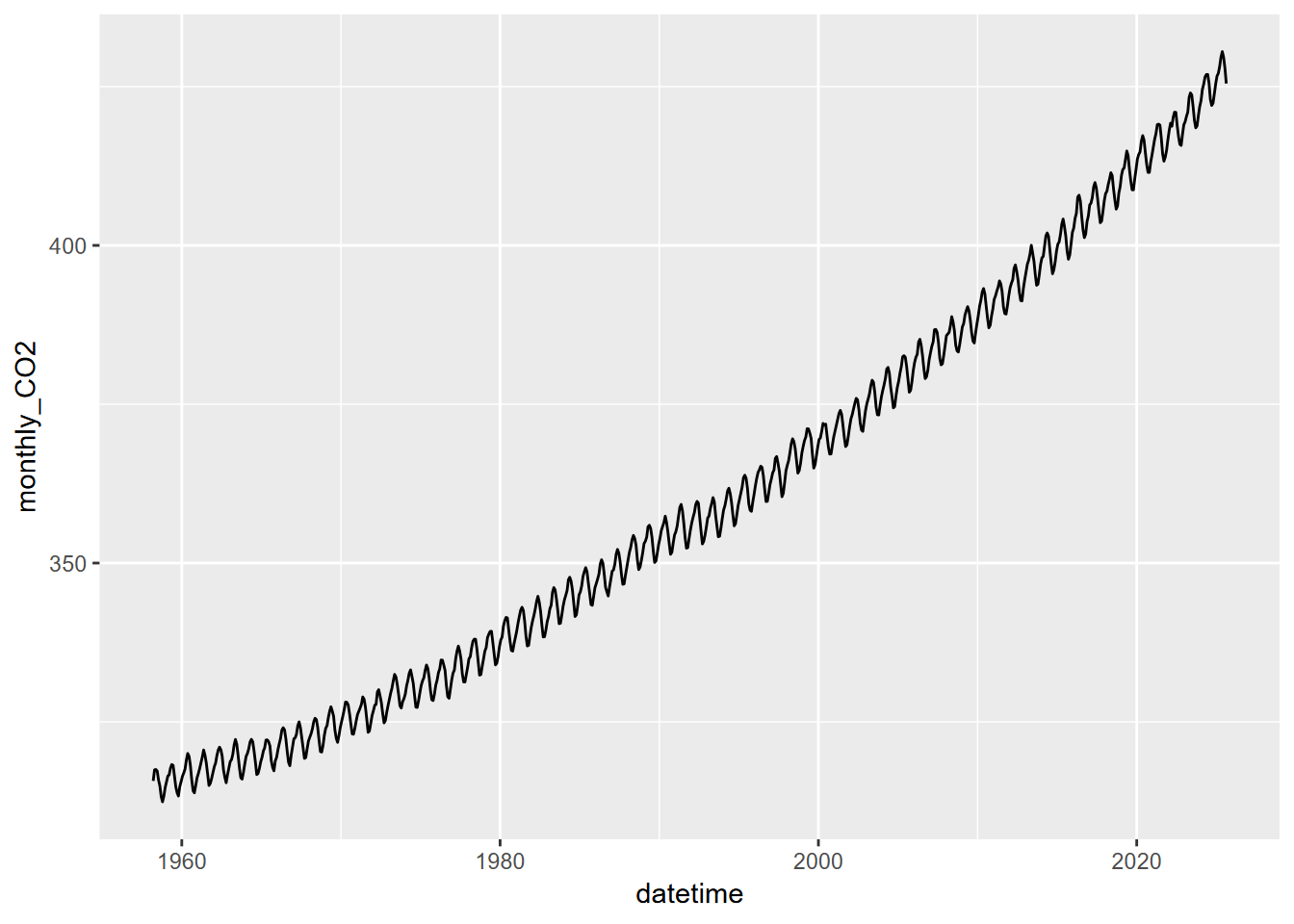Figure 11.2: Original February temperature data The prediction map library(tmap) tm_shape(hillsh, bbox=bounds) + tm_raster(palette="-Greys", style="cont", legend.show=F) + tm_shape(st_make_valid(CA_counties)) + tm_borders() + tm_shape(ct) + tm_dots() + tm_text("AREANAME", size=0.5, just=c("left","top")) + tm_shape(sierra) + tm_symbols(col="predict", palette="-RdBu", size=0.5) + tm_layout(legend.position=c("right","top")) + tm_graticules(lines=F) + tm_layout(main.title=eqn, main.title.position = c("right"), main.title.size = 0.8)Figure 11.3: Temperature predicted by elevation model We can also use the model to predict temperatures from a raster of elevations, which should match the elevations at the weather stations, allowing us to predict temperature for the rest of the study area. We should have more confidence in the area (the Sierra Nevada and nearby areas) actually sampled by the stations, so we’ll crop with the extent covering that area (though this will include more coastal areas that are likely to differ in February temperatures from patterns observed in the area sampled). We’ll use GCS elevation raster data. library(igisci) library(tmap); library(terra) elev <- rast(ex("CA/ca_elev_WGS84.tif")) elevSierra <- crop(elev, ext(-122, -118, 35, 41)) # GCS b0 <- coef(modelElev) b1 <- coef(modelElev) tempSierra <- b0 + b1 * elevSierra names(tempSierra) = "Predicted Temperature" #tempCA <- b0 + b1 * elev tm_shape(tempSierra) + tm_raster(palette="-RdBu") + tm_graticules(lines=F) + tm_legend(position = c("right", "top")) + tm_shape(st_make_valid(CA_counties)) + tm_borders() + tm_shape(ct) + tm_dots() + tm_text("AREANAME", size=0.5, just=c("left","top")) + tm_layout(main.title=eqn, main.title.position = c("right"), main.title.size = 0.7)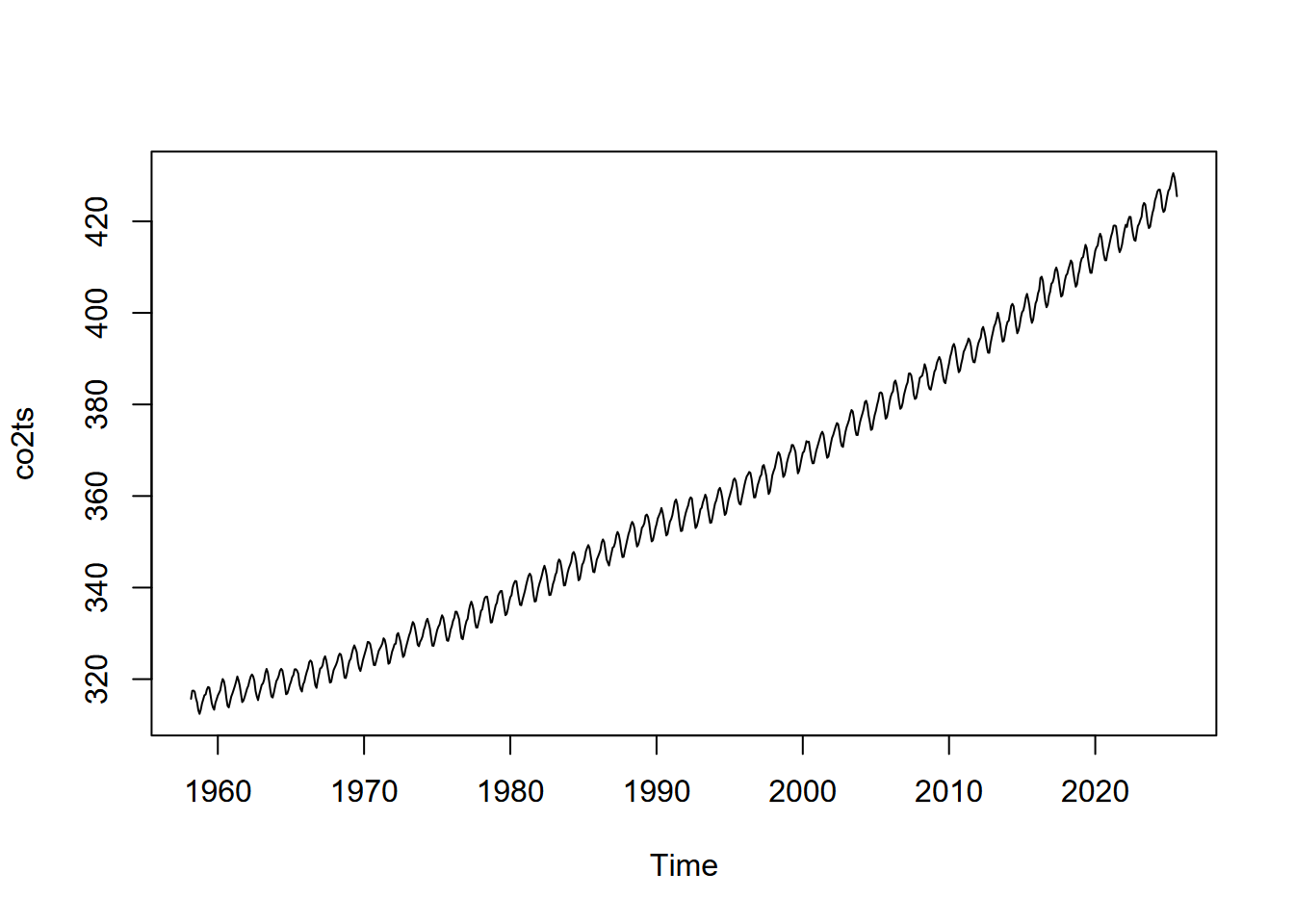Figure 11.4: Temperature predicted by elevation raster The residuals library(tmap) tm_shape(hillsh, bbox=bounds) + tm_raster(palette="-Greys", style="cont", legend.show=F) + tm_shape(st_make_valid(CA_counties)) + tm_borders() + tm_shape(ct) + tm_dots() + tm_text("AREANAME", size=0.5, just=c("left","top")) + tm_shape(sierra) + tm_symbols(col="resid", palette="-RdBu", size=0.5) + tm_layout(legend.position=c("right","top"), main.title = paste("e from",eqn,"+ e"), main.title.size = 0.7, main.title.position = "right") + tm_graticules(lines=F)Figure 11.5: Residuals Statistically, if the residuals from regression are spatially autocorrelated, we should look for patterns in the residuals to find other explanatory variables. However we can visually see, based upon the pattern of the residuals, that there’s at least one important explanatory variable not included in the model: latitude. We’ll leave that for you to look at in the exercises. ### 11.3.2 Analysis of Covariance Analysis of Covariance, or ANCOVA, has the same purpose as Analysis of Variance that we looked at under tests earlier, but also takes into account the influence of other variables called covariates. In this way, analysis of covariance combines a linear model with an analysis of variance. “Are water samples from streams draining sandstone, limestone, and shale different based on carbonate solutes, while taking into account elevation?” Response variable is modeled from the factor (ANOVA) plus the covariate (regression) • ANOVA: TH ~ rocktype $where TH is total hardness, a measure of carbonate solutes$ • Regression: TH ~ elevation • ANCOVA: TH ~ rocktype + elevation • Yet shouldn’t involve interaction between rocktype (factor) and elevation (covariate): Example: stream types distinguished by discharge and slope Three common river types are meandering, braided and anastomosed. For each, their slope varies by bankfull discharge in a relationship that looks something like:Figure 11.6: Slope by Q, river types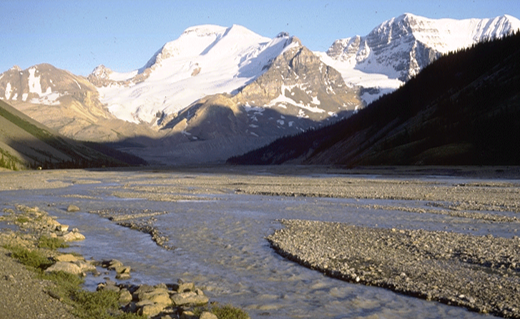Figure 11.7: Braided river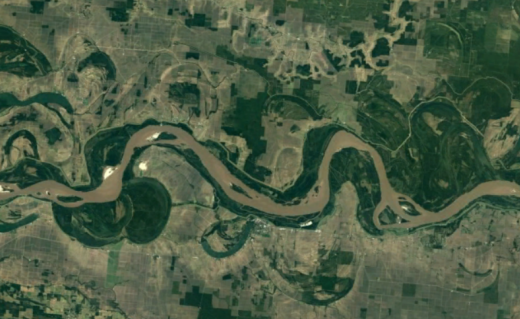Figure 11.8: Meandering river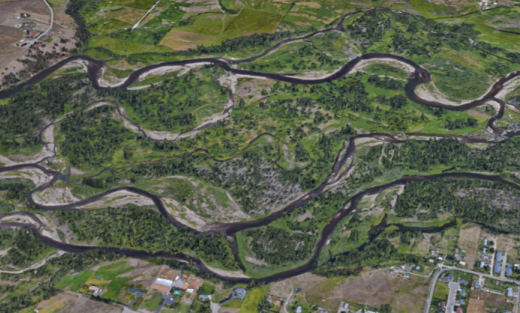Figure 11.9: Anastomosed river Requirements: • No relationship between discharge (covariate) and channel type (factor). • Another interpretation: the slope of the relationship between the covariate and response variable is about the same for each group; only the intercept differs. Assumes parallel slopes. log10(S) ~ strtype * log10(Q) … interaction between covariate and factor log10(S) ~ strtype + log10(Q) … no interaction, parallel slopes If models are not significantly different, remove interaction term due to parsimony, and satisfies this ANCOVA requirement. library(tidyverse) csvPath <- system.file("extdata","streams.csv", package="igisci") streams <- read_csv(csvPath) streams$strtype <- factor(streams$type, labels=c("Anastomosing","Braided","Meandering")) summary(streams) ## type Q S strtype ## Length:41 Min. : 6 Min. :0.000011 Anastomosing: 8 ## Class :character 1st Qu.: 15 1st Qu.:0.000100 Braided :12 ## Mode :character Median : 40 Median :0.000700 Meandering :21 ## Mean : 4159 Mean :0.001737 ## 3rd Qu.: 550 3rd Qu.:0.002800 ## Max. :100000 Max. :0.009500 ggplot(streams, aes(Q, S, color=strtype)) + geom_point()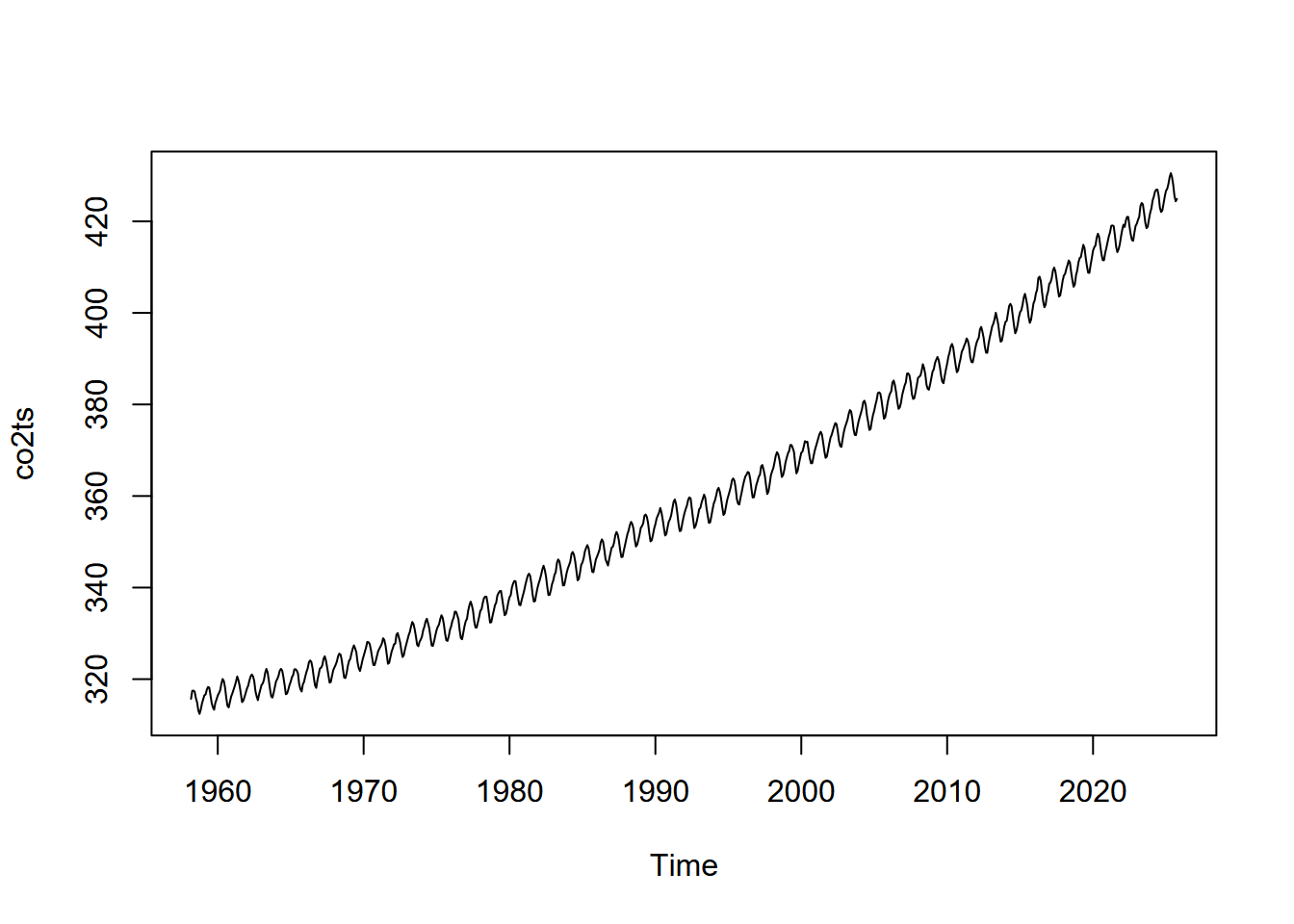Figure 11.10: Q vs S: needs log scaling library(scales) # needed for the trans_format function below ggplot(streams, aes(Q, S, color=strtype)) + geom_point() + geom_smooth(method="lm", se = FALSE) + scale_x_continuous(trans=log10_trans(), labels = trans_format("log10", math_format(10^.x))) + scale_y_continuous(trans=log10_trans(), labels = trans_format("log10", math_format(10^.x)))Figure 11.11: log scaled Q vs S with stream type ancova = lm(log10(S)~strtype*log10(Q), data=streams) summary(ancova) ## ## Call: ## lm(formula = log10(S) ~ strtype * log10(Q), data = streams) ## ## Residuals: ## Min 1Q Median 3Q Max ## -0.63636 -0.13903 -0.00032 0.12652 0.60750 ## ## Coefficients: ## Estimate Std. Error t value Pr(>|t|) ## (Intercept) -3.91819 0.31094 -12.601 1.45e-14 *** ## strtypeBraided 2.20085 0.35383 6.220 3.96e-07 *** ## strtypeMeandering 1.63479 0.33153 4.931 1.98e-05 *** ## log10(Q) -0.43537 0.18073 -2.409 0.0214 * ## strtypeBraided:log10(Q) -0.01488 0.19102 -0.078 0.9384 ## strtypeMeandering:log10(Q) 0.05183 0.18748 0.276 0.7838 ## --- ## Signif. codes: 0 '***' 0.001 '**' 0.01 '*' 0.05 '.' 0.1 ' ' 1 ## ## Residual standard error: 0.2656 on 35 degrees of freedom ## Multiple R-squared: 0.9154, Adjusted R-squared: 0.9033 ## F-statistic: 75.73 on 5 and 35 DF, p-value: < 2.2e-16 anova(ancova) ## Analysis of Variance Table ## ## Response: log10(S) ## Df Sum Sq Mean Sq F value Pr(>F) ## strtype 2 18.3914 9.1957 130.3650 < 2.2e-16 *** ## log10(Q) 1 8.2658 8.2658 117.1821 1.023e-12 *** ## strtype:log10(Q) 2 0.0511 0.0255 0.3619 0.6989 ## Residuals 35 2.4688 0.0705 ## --- ## Signif. codes: 0 '***' 0.001 '**' 0.01 '*' 0.05 '.' 0.1 ' ' 1 # Now an additive model, which does not have that interaction ancova2 = lm(log10(S)~strtype+log10(Q), data=streams) anova(ancova2) ## Analysis of Variance Table ## ## Response: log10(S) ## Df Sum Sq Mean Sq F value Pr(>F) ## strtype 2 18.3914 9.1957 135.02 < 2.2e-16 *** ## log10(Q) 1 8.2658 8.2658 121.37 3.07e-13 *** ## Residuals 37 2.5199 0.0681 ## --- ## Signif. codes: 0 '***' 0.001 '**' 0.01 '*' 0.05 '.' 0.1 ' ' 1 anova(ancova,ancova2)  ## Analysis of Variance Table ## ## Model 1: log10(S) ~ strtype * log10(Q) ## Model 2: log10(S) ~ strtype + log10(Q) ## Res.Df RSS Df Sum of Sq F Pr(>F) ## 1 35 2.4688 ## 2 37 2.5199 -2 -0.051051 0.3619 0.6989  # not significantly different, so model simplification is justified # Now we remove the strtype term ancova3 = update(ancova2, ~ . - strtype) anova(ancova2,ancova3)  ## Analysis of Variance Table ## ## Model 1: log10(S) ~ strtype + log10(Q) ## Model 2: log10(S) ~ log10(Q) ## Res.Df RSS Df Sum of Sq F Pr(>F) ## 1 37 2.5199 ## 2 39 25.5099 -2 -22.99 168.78 < 2.2e-16 *** ## --- ## Signif. codes: 0 '***' 0.001 '**' 0.01 '*' 0.05 '.' 0.1 ' ' 1  # Goes too far. Removing the strtype creates a significantly different model step(ancova) ## Start: AIC=-103.2 ## log10(S) ~ strtype * log10(Q) ## ## Df Sum of Sq RSS AIC ## - strtype:log10(Q) 2 0.051051 2.5199 -106.36 ## <none> 2.4688 -103.20 ## ## Step: AIC=-106.36 ## log10(S) ~ strtype + log10(Q) ## ## Df Sum of Sq RSS AIC ## <none> 2.5199 -106.364 ## - log10(Q) 1 8.2658 10.7857 -48.750 ## - strtype 2 22.9901 25.5099 -15.455 ## ## Call: ## lm(formula = log10(S) ~ strtype + log10(Q), data = streams) ## ## Coefficients: ## (Intercept) strtypeBraided strtypeMeandering log10(Q) ## -3.9583 2.1453 1.7294 -0.4109 ANOVA & ANCOVA are applications of a linear model. • Uses lm in R • Response variable is continuous, assumed normally distributed mymodel = lm(log10(S) ~ strtype + log10(Q)) • The linear model, with categorical explanatory variable + covariate anova(mymodel) • Displays the Analysis of Variance table from the linear model ## 11.4 Generalized Linear Model (GLM) The glm in R allows you to work with various types of data using various distributions, described as families such as: • gaussian : normal distribution – what is used with lm • binomial : logit – used with probabilities. • poisson : for counts. Commonly used for species counts. • see help(glm) for other examples ### 11.4.1 Binomial family: Logistic GLM with streams When the glm family is binomial, we might do what’s sometimes called logistic regression where we look at the probability of a categorical value (might be a species occurrence, for instance) given various independent variables. Let’s try this with the streams data we just used for analysis of covariance, to predict the probability of a particular stream type from slope and discharge: library(igisci) library(tidyverse) streams <- read_csv(ex("streams.csv")) streams$strtype <- factor(streams$type, labels=c("Anastomosing","Braided","Meandering")) For logistic regression, we need probability data, so locations where we know the probability of something being true. If you think about it, that’s pretty difficult to know for an observation, but we might know whether something is true or not, like we observed the phenomenon or not, and so we just assign a probability of 1 if something is observed, and 0 if it’s not. Since in the case of the stream types, we really have three different probabilities: whether it’s true that the stream is anastomosing, whether it’s braided and whether it’s meandering, so we’ll create three dummy variables for that with values of 1 or 0: if it’s braided, the value for braided is 1, otherwise it’s 0; the same applies to all three dummy variables: streams <- streams %>% mutate(braided = ifelse(type == "B", 1, 0), meandering = ifelse(type == "M", 1, 0), anastomosed = ifelse(type == "A", 1, 0)) streams ## # A tibble: 41 x 7 ## type Q S strtype braided meandering anastomosed ## <chr> <dbl> <dbl> <fct> <dbl> <dbl> <dbl> ## 1 B 9.9 0.0045 Braided 1 0 0 ## 2 B 11 0.007 Braided 1 0 0 ## 3 B 16 0.0068 Braided 1 0 0 ## 4 B 25 0.0042 Braided 1 0 0 ## 5 B 40 0.0028 Braided 1 0 0 ## 6 B 150 0.0041 Braided 1 0 0 ## 7 B 190 0.002 Braided 1 0 0 ## 8 B 550 0.0007 Braided 1 0 0 ## 9 B 1100 0.00055 Braided 1 0 0 ## 10 B 7100 0.00042 Braided 1 0 0 ## # ... with 31 more rows Then we can run the logistic regression on each one: summary(glm(braided ~ log(Q) + log(S), family=binomial, data=streams)) ## ## Call: ## glm(formula = braided ~ log(Q) + log(S), family = binomial, data = streams) ## ## Deviance Residuals: ## Min 1Q Median 3Q Max ## -2.00386 -0.49007 -0.00961 0.15789 1.58088 ## ## Coefficients: ## Estimate Std. Error z value Pr(>|z|) ## (Intercept) 17.5853 6.7443 2.607 0.00912 ** ## log(Q) 2.0940 0.8135 2.574 0.01005 * ## log(S) 4.3115 1.6173 2.666 0.00768 ** ## --- ## Signif. codes: 0 '***' 0.001 '**' 0.01 '*' 0.05 '.' 0.1 ' ' 1 ## ## (Dispersion parameter for binomial family taken to be 1) ## ## Null deviance: 49.572 on 40 degrees of freedom ## Residual deviance: 23.484 on 38 degrees of freedom ## AIC: 29.484 ## ## Number of Fisher Scoring iterations: 8 summary(glm(meandering ~ log(Q) + log(S), family=binomial, data=streams)) ## ## Call: ## glm(formula = meandering ~ log(Q) + log(S), family = binomial, ## data = streams) ## ## Deviance Residuals: ## Min 1Q Median 3Q Max ## -1.5469 -1.2515 0.8197 1.1073 1.3072 ## ## Coefficients: ## Estimate Std. Error z value Pr(>|z|) ## (Intercept) 2.43255 1.38078 1.762 0.0781 . ## log(Q) 0.04984 0.13415 0.372 0.7103 ## log(S) 0.34750 0.19471 1.785 0.0743 . ## --- ## Signif. codes: 0 '***' 0.001 '**' 0.01 '*' 0.05 '.' 0.1 ' ' 1 ## ## (Dispersion parameter for binomial family taken to be 1) ## ## Null deviance: 56.814 on 40 degrees of freedom ## Residual deviance: 53.074 on 38 degrees of freedom ## AIC: 59.074 ## ## Number of Fisher Scoring iterations: 4 summary(glm(anastomosed ~ log(Q) + log(S), family=binomial, data=streams)) ## ## Call: ## glm(formula = anastomosed ~ log(Q) + log(S), family = binomial, ## data = streams) ## ## Deviance Residuals: ## Min 1Q Median 3Q Max ## -2.815e-05 -2.110e-08 -2.110e-08 -2.110e-08 2.244e-05 ## ## Coefficients: ## Estimate Std. Error z value Pr(>|z|) ## (Intercept) -176.09 786410.69 0 1 ## log(Q) -11.81 77224.35 0 1 ## log(S) -24.18 70417.40 0 1 ## ## (Dispersion parameter for binomial family taken to be 1) ## ## Null deviance: 4.0472e+01 on 40 degrees of freedom ## Residual deviance: 1.3027e-09 on 38 degrees of freedom ## AIC: 6 ## ## Number of Fisher Scoring iterations: 25 So what we can see from this admittedly small sample is that: • Predicting braided character from Q and S works pretty well, distinguishing these characteristics from the characteristics of all other streams $braided==0$ • Predicting anastomosed or meandering however isn’t significant. We really need a larger sample size, and North American geomorphology has recently discovered the “anabranching” stream type (of which anastomosed is a type) that had been long recognized in Australia, and its characteristics range across meandering at least, so muddying the waters… ### 11.4.2 Logistic landslide model With its active tectonics, steep slopes and relatively common intense rain events during the winter season, coastal California has a propensity for landslides. Pacifica has been the focus of many landslide studies by the USGS and others, with events ranging from coastal erosion influenced by wave action to landslides and debris flows on steep hillslopes driven by positive pore pressure ().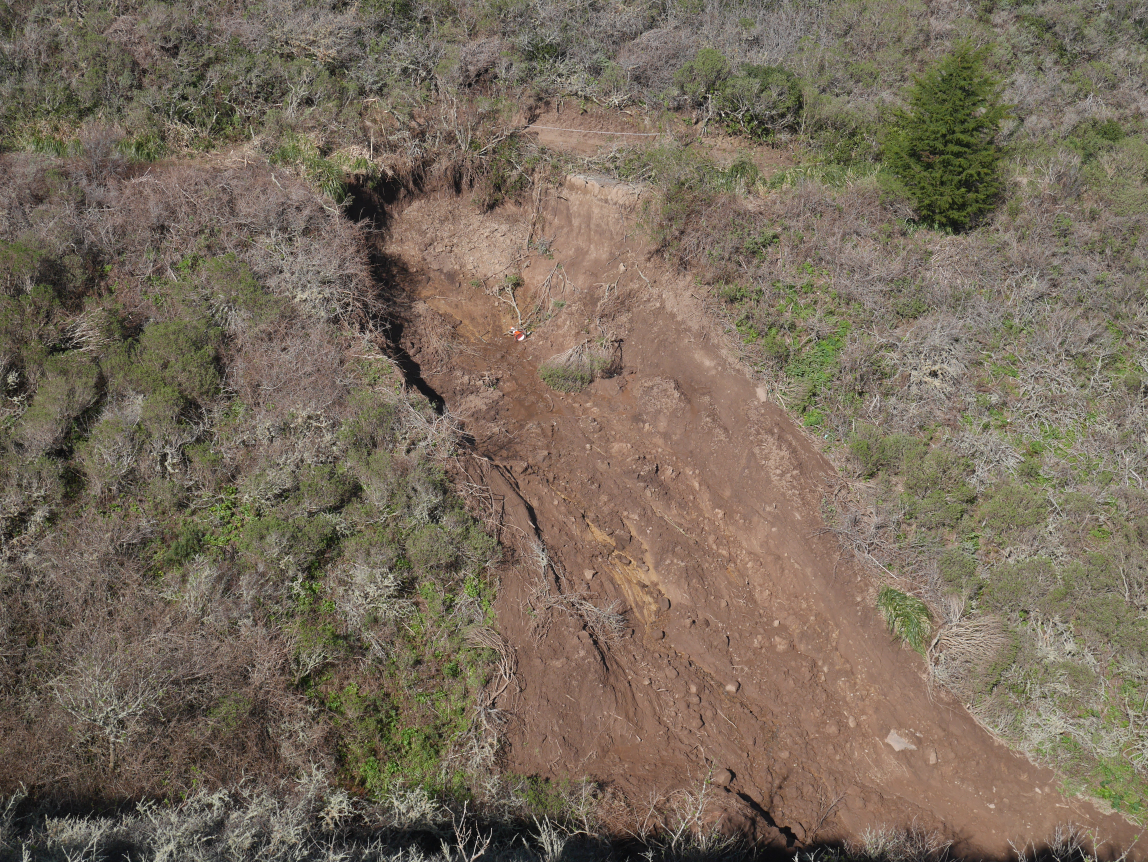Figure 11.12: Landslide in San Pedro Creek watershed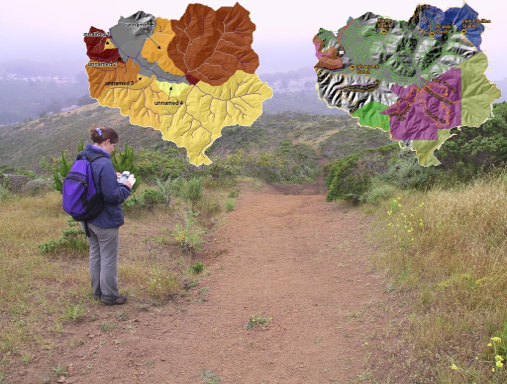Figure 11.13: Sediment source analysis Also see the relevant section in the San Pedro Creek Virtual Fieldtrip story map: . Landslides detected in San Pedro Creek Watershed in Pacifica, CA, are in the SanPedro folder of extdata. Landslides were detected on aerial photography from 1941, 1955, 1975, 1983, and 1997, and confirmed in the field during a study in the early 2000’s (Sims (2004), ). We’ll create a logistic regression to compare landslide probability to various environmental factors. We’ll use elevation, slope and other derivatives, distance to streams, distance to trails, and the results of a physical model predicting slope stability as a stability index (SI), using slope and soil transmissivity data. library(igisci); library(sf); library(tidyverse); library(terra) slides <- st_read(ex("SanPedro/slideCentroids.shp")) %>% mutate(IsSlide = 1) slides$Visible <- factor(slides$Visible) trails <- st_read(ex("SanPedro/trails.shp")) streams <- st_read(ex("SanPedro/streams.shp")) wshed <- st_read(ex("SanPedro/SPCWatershed.shp")) ggplot(data=slides) + geom_sf(aes(col=Visible)) + scale_color_brewer(palette="Spectral") + geom_sf(data=streams, col="blue") + geom_sf(data=wshed, fill=NA)Figure 11.14: Landslides in San Pedro Creek watershed At this point, we have data for occurrences of landslides, and so for each point we assign the dummy variable IsSlide = 1. We don’t have any absence data, but we can create some using random points and assign them IsSlide = 0. It would probably be a good idea to avoid areas very close to the occurrences, but we’ll just put random points everywhere and just remove the ones that don’t have SI data. For the random locations, we’ll set a seed so for the book the results will stay the same; and if we’re good with that since it doesn’t really matter what seed we use, we might as well use the answer to everything: 42. library(terra) elev <- rast(ex("SanPedro/dem.tif")) SI <- rast(ex("SanPedro/SI.tif")) npts <- length(slides$Visible) * 2 # up it a little to get enough after clip
set.seed(42)
x <- runif(npts, min=xmin(elev), max=xmax(elev))
y <- runif(npts, min=ymin(elev), max=ymax(elev))
rsampsRaw <- st_as_sf(data.frame(x,y), coords = c("x","y"), crs=crs(elev))
ggplot(data=rsampsRaw) + geom_sf(size=0.5) +
geom_sf(data=streams, col="blue") +
geom_sf(data=wshed, fill=NA)Figure 11.15: Raw random points

Now we have twice as many random points as we have landslides, within the rectangular extend of the watershed, as defined by the elevation raster. We doubled these because we need to exclude some points, yet still have enough to be roughly as many random points as slides. We’ll exclude:

• all points outside the watershed
• points within 100 m of the landslide occurrences, the distance based on knowledge of the grain of landscape features (like spurs and swales) that might influence landslide occurrence
slideBuff <- st_buffer(slides, 100)
wshedNoSlide <- st_difference(wshed, st_union(slideBuff))

The 100 m buffer excludes all areas around all landslides in the data set. We’re going to run the model on specific years, but the best selection of landslide absences will be to exclude areas around all landslides we know of.

ggplot(data=slides) + geom_sf(aes(col=Visible)) +
scale_color_brewer(palette="Spectral") +
geom_sf(data=streams, col="blue") +
geom_sf(data=wshedNoSlide, fill=NA)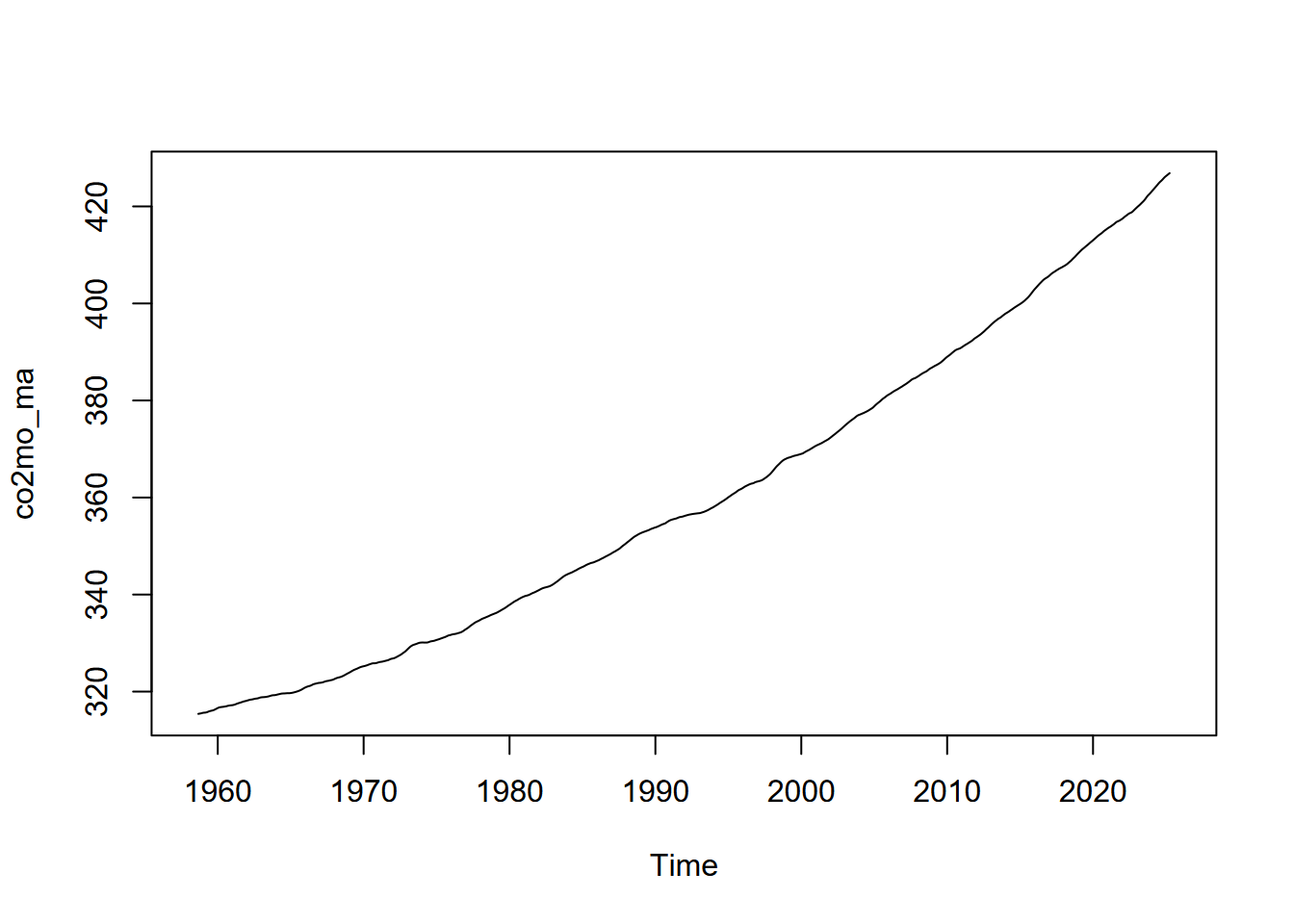Figure 11.16: Landslides and buffers to exclude from random points

rsamps <- st_intersection(rsampsRaw,wshedNoSlide) %>%
mutate(IsSlide = 0)
allPts <- bind_rows(slides, rsamps)
ggplot(data=allPts) + geom_sf(aes(col=IsSlide)) +
geom_sf(data=streams, col="blue") +
geom_sf(data=wshed, fill=NA)Figure 11.17: Landslides and random points (excluded from slide buffers)

Now we’ll derive some environmental variables as rasters and extract their values to the slide/noslide point data set. We’ll use the shade function to create an exposure raster with the sun at the average noon angle from the south. Note the use of dplyr::rename to create meaningful variable names for rasters that had names like "lyr1" (who knows why?) which I determined by running it without the rename and seeing what it produced.

slidesV <- vect(allPts)
strms <- rasterize(vect(streams),elev)
tr <- rasterize(vect(trails),elev)
stD <- distance(strms); names(stD) = "stD"
trD <- distance(tr); names(trD) = "trD"
slope <- terrain(elev, v="slope")
curv <- terrain(slope, v="slope")
aspect <- terrain(elev, v="aspect")
elev_ex <- terra::extract(elev, slidesV) %>% rename(elev=dem) %>% dplyr::select(-ID)
SI_ex <- terra::extract(SI, slidesV) %>% dplyr::select(-ID)
slope_ex <- terra::extract(slope, slidesV) %>% dplyr::select(-ID)
curv_ex <- terra::extract(curv, slidesV) %>% rename(curv = slope) %>% dplyr::select(-ID)
exposure_ex <- terra::extract(exposure, slidesV) %>% rename(exposure=lyr1) %>% dplyr::select(-ID)
stD_ex <- terra::extract(stD, slidesV) %>% dplyr::select(-ID)
trD_ex <- terra::extract(trD, slidesV) %>% dplyr::select(-ID)
slidePts <- cbind(allPts,elev_ex,SI_ex,slope_ex,curv_ex,exposure_ex,stD_ex,trD_ex)
slidesData <- as.data.frame(slidePts) %>%
filter(!is.nan(SI)) %>% st_as_sf(crs=st_crs(slides))

We’ll filter for just the slides in the 1983 imagery, and for the model also include all of the random points (detected with NA values for Visible):

slides83 <- slidesData %>% filter(Visible == 1983)
slides83plusr <- slidesData %>% filter(Visible == 1983 | is.na(Visible))

The logistic regression in the glm model uses the binomial family:

summary(glm(IsSlide ~ SI + curv + elev + slope + exposure + stD + trD,
family=binomial, data=slides83plusr))
##
## Call:
## glm(formula = IsSlide ~ SI + curv + elev + slope + exposure +
##     stD + trD, family = binomial, data = slides83plusr)
##
## Deviance Residuals:
##     Min       1Q   Median       3Q      Max
## -2.3819  -0.5882  -0.0002   0.7378   3.9146
##
## Coefficients:
##               Estimate Std. Error z value Pr(>|z|)
## (Intercept)  0.1426071  1.0618610   0.134  0.89317
## SI          -1.8190286  0.3639202  -4.998 5.78e-07 ***
## curv         0.0059237  0.0113657   0.521  0.60223
## elev        -0.0025050  0.0014398  -1.740  0.08190 .
## slope        0.0623201  0.0211010   2.953  0.00314 **
## exposure     2.6971912  0.6603844   4.084 4.42e-05 ***
## stD         -0.0052639  0.0019765  -2.663  0.00774 **
## trD         -0.0020730  0.0006532  -3.174  0.00150 **
## ---
## Signif. codes:  0 '***' 0.001 '**' 0.01 '*' 0.05 '.' 0.1 ' ' 1
##
## (Dispersion parameter for binomial family taken to be 1)
##
##     Null deviance: 668.29  on 483  degrees of freedom
## Residual deviance: 402.90  on 476  degrees of freedom
## AIC: 418.9
##
## Number of Fisher Scoring iterations: 8

So while we might want to make sure that we’ve picked the right variables, some conclusions from this might be that stability index (SI) is not surprisingly a strong predictor of landslides, but also that other than elevation and curvature, the other variables are also significant.

#### 11.4.2.1 Map the logistic result

Should be able to, just need to use the formula for the model, which in our case will have 6 explanatory variables SI ($$X_1$$), elev ($$X_2$$), slope ($$X_3$$), exposure ($$X_4$$), stD ($$X_5$$) and trD ($$X_6$$): $p = \frac{e^{b_0+b_1X_1+b_2X_2+b_3X_3+b_4X_4+b_5X_5}}{1+e^{b_0+b_1X_1+b_2X_2+b_3X_3+b_4X_4+b_5X_5}}$

Then we retrieve the coefficients with coef() and use map algebra to create the prediction map, including dots for the landslides actually observed in 1983:

library(tmap)
SI_stD_model <- glm(IsSlide ~ SI + slope + exposure + stD + trD, family=binomial, data=slides83plusr)
b0 <- coef(SI_stD_model)
b1 <- coef(SI_stD_model)
b2 <- coef(SI_stD_model)
b3 <- coef(SI_stD_model)
b4 <- coef(SI_stD_model)
b5 <- coef(SI_stD_model)
predictionMap <- (exp(b0+b1*SI+b2*slope+b3*exposure+b4*stD+b5*trD))/
(1 + exp(b0+b1*SI+b2*slope+b3*exposure+b4*stD+b5*trD))
names(predictionMap) <- "slide_probability_1983"
tm_shape(predictionMap) + tm_raster() +
tm_shape(streams) + tm_lines(col="blue") +
tm_shape(wshed) + tm_borders(col="black") +
tm_shape(slides83) + tm_dots(col="green") +
tm_layout(title="Predicted by SI, slope, exposure, streamD, trailD") +
tm_graticules(lines=F)Figure 11.18: Logistic model prediction of 1983 landslide probability

### 11.4.3 Poisson regression

Poission regression (family=poisson in glm) is for analyzing counts. You might use this to determine which variables are significant predictors, or you might want to see where or when counts are particularly high, or higher than expected. Or it might be applied to compare groupings. For example, if instead of looking at differences among rock types in Sinking Cove based on chemical properties in water samples or measurements (where we applied ANOVA), we looked at differences in fish counts, that might be an application of poisson regression, as long as other data assumptions hold.

#### 11.4.3.1 Seabird Poisson Model

This model is further developed in a case study where we’ll map a prediction, but we’ll look at the basics for applying a poisson family model in glm: seabird observations. The data is potentially suitable since it’s counts with known time intervals of observation effort and initial review of the data suggests that the mean counts is reasonably similar to the variance. The data were collected through the ACCESS program () and a thorough analysis provided by .

library(igisci); library(sf); library(tidyverse); library(tmap)
library(maptiles)
transects <- st_read(ex("SFmarine/transects.shp"))

One of the seabirds studies, the black-footed albatross, has the lowest counts so a relatively rare bird, and studies of its distribution may be informative. We’ll look at counts collected during transect cruises in July 2006.

tmap_mode("plot")
oceanBase <- get_tiles(transects, provider="Esri.OceanBasemap")
transJul2006 <- transects %>% filter(month==7 & year==2006 & avg_tem>0 & avg_sal>0 & avg_fluo>0)
tm_shape(oceanBase) + tm_rgb() +
tm_shape(transJul2006) + tm_symbols(col="bfal", size="bfal")Figure 11.19: Black-footed albatross counts, July 2006

Prior studies have suggested that temperature, salinity, fluorescence, depth and various distances might be good explanatory variables to use to look at spatial patterns, so we’ll use these in the model.

summary(glm(bfal~avg_tem+avg_sal+avg_fluo+avg_dep+dist_land+dist_isla+dist_200m+dist_cord, data=transJul2006,family=poisson))
##
## Call:
## glm(formula = bfal ~ avg_tem + avg_sal + avg_fluo + avg_dep +
##     dist_land + dist_isla + dist_200m + dist_cord, family = poisson,
##     data = transJul2006)
##
## Deviance Residuals:
##     Min       1Q   Median       3Q      Max
## -1.2935  -0.4646  -0.2445  -0.0945   4.7760
##
## Coefficients:
##               Estimate Std. Error z value Pr(>|z|)
## (Intercept) -1.754e+02  7.032e+01  -2.495  0.01260 *
## avg_tem      7.341e-01  3.479e-01   2.110  0.03482 *
## avg_sal      5.202e+00  2.097e+00   2.480  0.01312 *
## avg_fluo    -6.978e-01  1.217e+00  -0.574  0.56626
## avg_dep     -3.561e-03  8.229e-04  -4.328 1.51e-05 ***
## dist_land   -2.197e-04  6.194e-05  -3.547  0.00039 ***
## dist_isla    1.372e-05  1.778e-05   0.771  0.44043
## dist_200m   -4.696e-04  1.037e-04  -4.528 5.95e-06 ***
## dist_cord   -5.213e-06  1.830e-05  -0.285  0.77570
## ---
## Signif. codes:  0 '***' 0.001 '**' 0.01 '*' 0.05 '.' 0.1 ' ' 1
##
## (Dispersion parameter for poisson family taken to be 1)
##
##     Null deviance: 152.14  on 206  degrees of freedom
## Residual deviance: 105.73  on 198  degrees of freedom
## AIC: 160.37
##
## Number of Fisher Scoring iterations: 7

We can see in the model coefficients table several predictive variables that appear to be significant, which we’ll explore further in the case study.

• avg_tem
• avg_sal
• avg_dep
• dist_land
• dist_200m

Note R will allow you to run glm on any family, but it’s up to you to make sure it’s appropriate. For example, if you provide a response variable that is not count data or doesn’t fit the requirements for a poisson model, R will create a result but it’s bogus.

## 11.5 Models Employing Machine Learning

Models using machine learning algorithms are commonly used in data science, fitting with its general exploratory and data-mining approach. There are many machine learning algorithms, and many resources for learning more about them, but they all share a basically black-box approach where a collection of variables are explored for patterns in input variables that help to explain a response variable. The latter is similar to more conventional statistical modeling describe above, with the difference being the machine learning approach – think of robots going through your data looking for connections.

We’ll explore a few machine learning methods (such as neural networks, random forests, and support vector machines) in a later chapter when we attempt to use them to classify satellite imagery, an important environmental application. As this application is in the spatial domain, we might want to look further into spatial statistical methods.

## 11.6 Exercise

1. Start by creating a new RStudio project “modeling” including a “data” folder we’ll use for the last question when you bring in your own data.

2. Add LATITUDE as a second independent variable after ELEVATION in the model predicting February temperatures. Note that you’ll need to change a setting in the st_as_sf to not remove LATITUDE and LONGITUDE, and then you’ll need to change the lm regression formula to include both ELEVATION + LATITUDE. First derive and display the model summary results, and then answer the questions – is the explanation better based on the $$r^2$$?

3. Now map the predictions and residuals.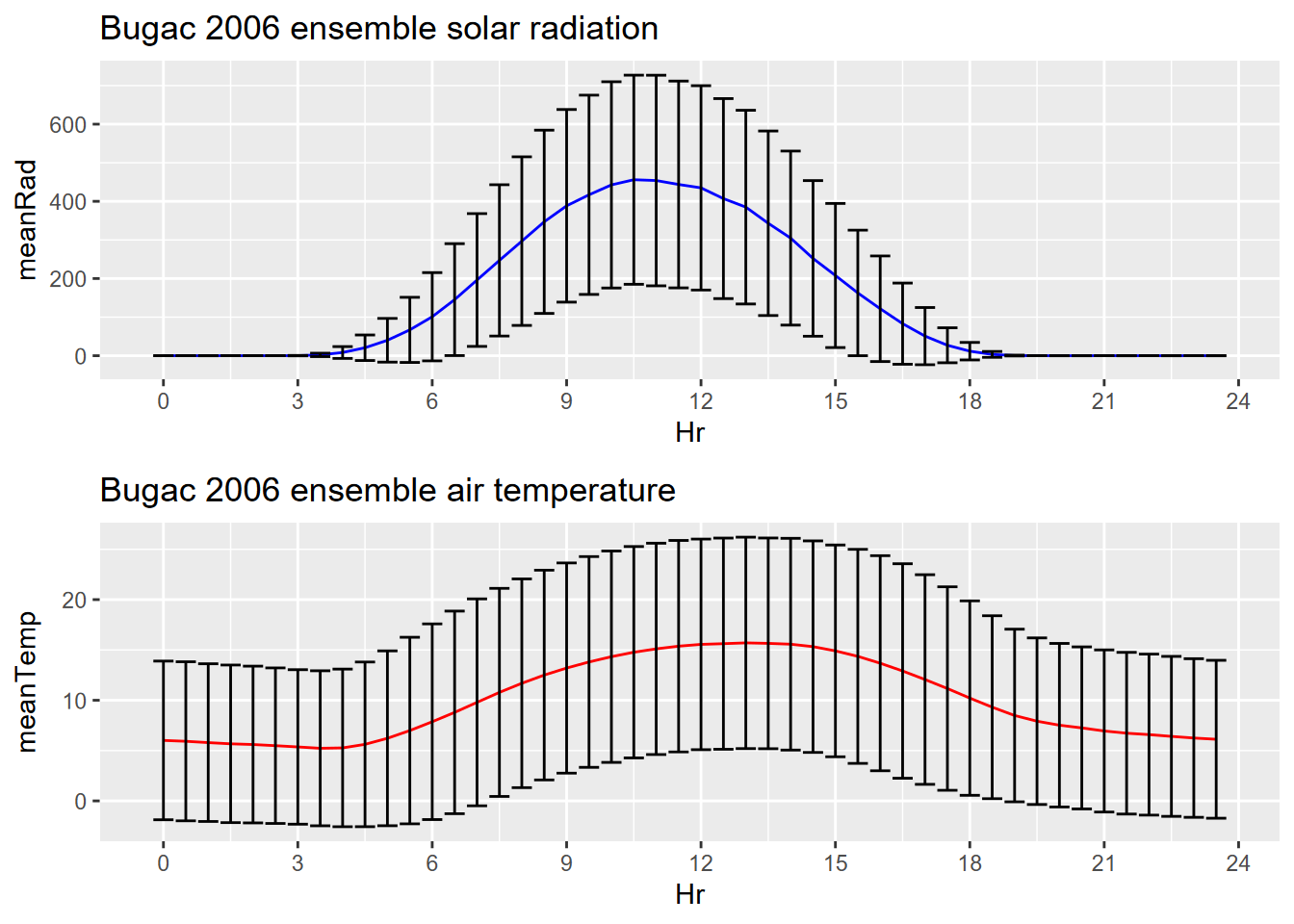Figure 11.20: Goal of Question 2

1. Can you see any potential for additional variables? What evidence might there be for localized effects?

2. Let’s build a prediction raster from the above model. Start by reading in the ca_elev_WGS84.tif raster as was done earlier, with the same crop, and again assigning it to elevSierra, and get the three coefficients b0, b1, and b2.

• Now use the following code to create a raster of the same dimensions as elevSierra, but with latitude assigned as z values to the cells:
lats <- yFromCell(elevSierra, 1:ncell(elevSierra))
latitude <- setValues(elevSierra,lats)
plot(latitude)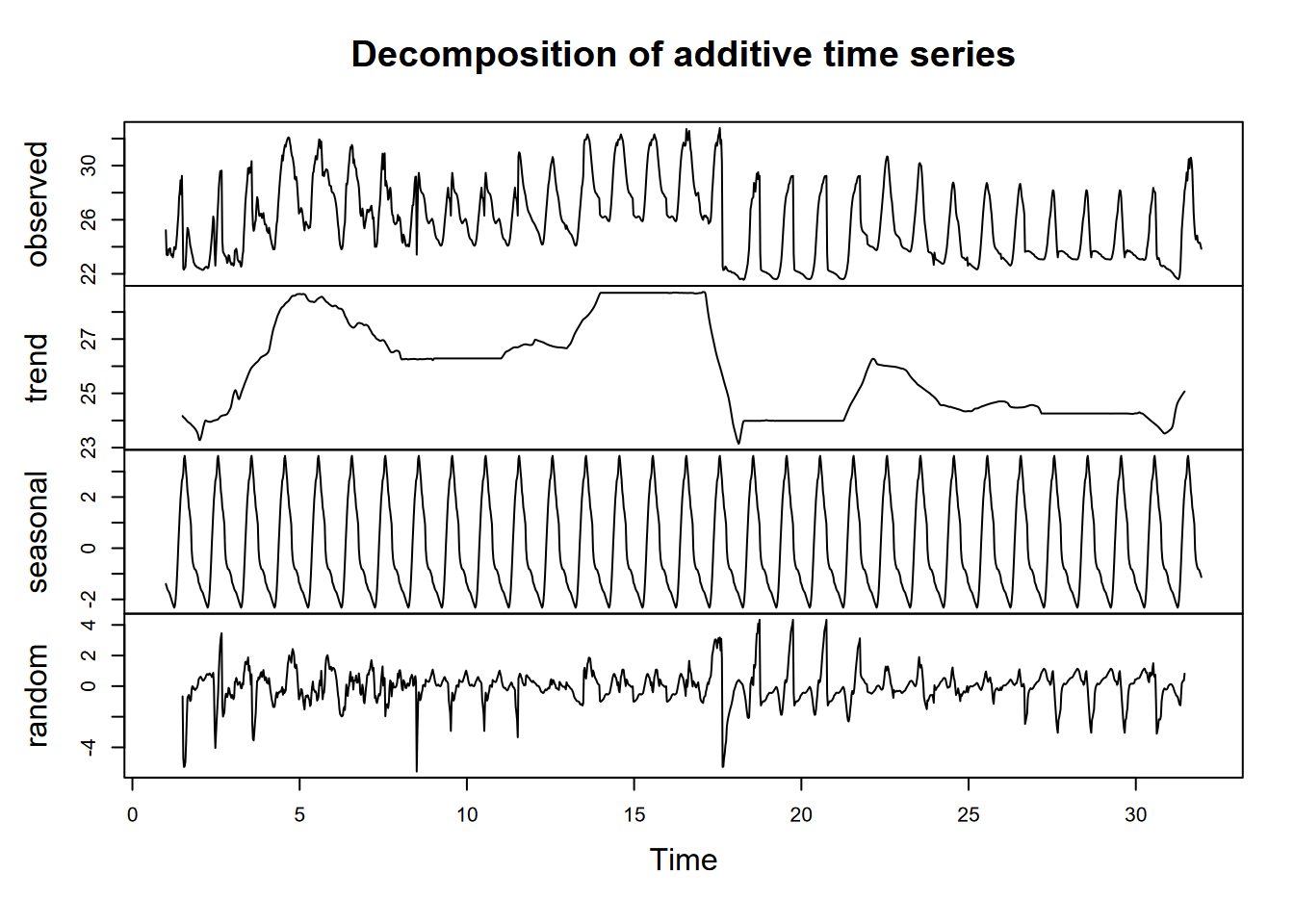Figure 11.21: Latitude raster

• Then build a raster prediction of temperature from the model and the two explanatory rasters.Figure 11.22: Goal of Question 4

1. Modify the code for the San Pedro Creek Watershed logistical model to look at 1975 landslides. Are the conclusions different?

2. Using your own data, that should have location information as well as other attributes, create a statistical model of your choice, and provide your assessment of the results, a prediction map, and where appropriate mapped residuals.

3. Optionally, using your own data, no location data needed, create a glm binomial model. If you don’t have anything obvious, consider doing something like the study of a pet dog panting based on temperature. https://bscheng.com/2016/10/23/logistic-regression-in-r/

### References

Applied California Current Ecosystem Studies. n.d. https://www.accessoceans.org.
Davis, Jerry. n.d. San Pedro Creek Watershed Virtual Fieldtrip: Story Map. https://storymaps.arcgis.com/stories/62705877a9f64ac5956a64230430c248.
Davis, Jerry, and Leonhard Blesius. 2015. “A Hybrid Physical and Maximum-Entropy Landslide Susceptibility Model.” Entropy 17 (6): 4271–92. https://www.mdpi.com/1099-4300/17/6/4271.
Ellen, Stephen D., and Gerald F. Wieczorek. 1988. Landslides, Floods, and Marine Effects of the Storm of January 3-5, 1982, in the San Francisco Bay Region, California. Vol. 1434. USGS. https://pubs.usgs.gov/pp/1988/1434/.
Hijmans, Robert J. n.d. Spatial Data Science. https://rspatial.org.
Sims, Stephanie. 2004. Hillslope Sediment Source Assessment of San Pedro Creek Watershed, California. https://geog.sfsu.edu/thesis/hillslope-sediment-source-assessment-san-pedro-creek-watershed-california.
Studwell, Anna, Ellen Hines, Meredith L. Elliott, Julie Howar, Barbara Holzman, Nadav Nur, and Jaime Jahncke. 2017. “Modeling Nonresident Seabird Foraging Distributions to Inform Ocean Zoning in Central California.” PLoS ONE. https://doi.org/10.1371/journal.pone.0169517.